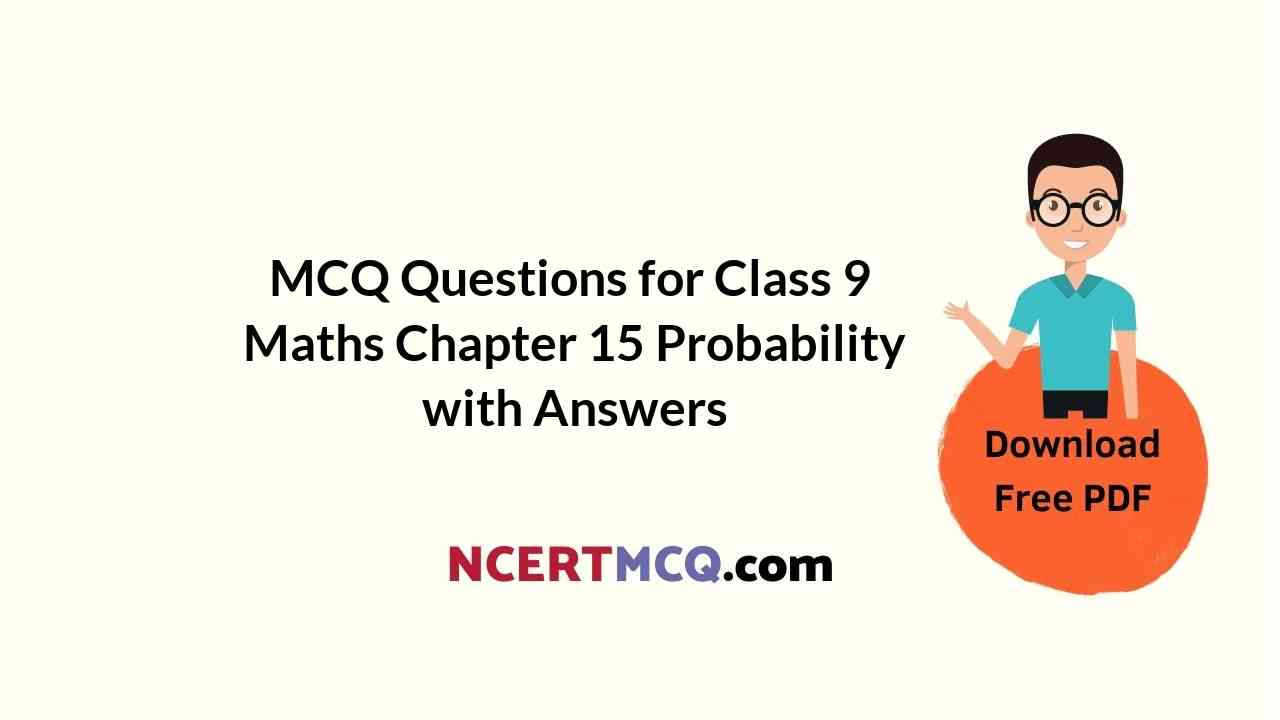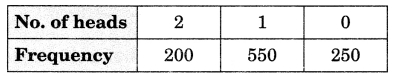Check the below Online Education NCERT MCQ Questions for Class 9 Maths Chapter 15 Probability with Answers Pdf free download. MCQ Questions for Class 9 Maths with Answers were prepared based on the latest exam pattern. We have provided Probability Class 10 Maths MCQs Questions with Answers to help students understand the concept very well. https://ncertmcq.com/mcq-questions-for-class-9-maths-with-answers/

Students can also refer to NCERT Solutions for Class 9 Maths Chapter 15 Probability for better exam preparation and score more marks.

## Online Education for Probability Class 9 MCQs Questions with Answers

Probability Class 9 MCQ Chapter 15 Question 1.
Which of the following cannot be the empirical probability of an event?
(a) $$\frac{2}{3}$$
(b) $$\frac{3}{2}$$
(c) 0
(d) 1

Answer: (b) $$\frac{3}{2}$$

Probability MCQ Class 9 Chapter 15 Question 2.
In a survey of 364 children aged 19-36 months, it was found that 91 liked to eat potato chips. If a child is selected at random, the probability that he/she does not like to eat potato chips is:
(a) 0.25
(b) 0.50
(c) 0.75
(d) 0.80

Class 9 Probability MCQ Chapter 15 Question 3.
In a sample study of 640 people, it was found that 512 people have a high school certificate. If a person is selected at random, the probability that the person has a high school certificate is:
(a) 0.5
(b) 0.6
(c) 0.7
(d) 0.8

MCQ On Probability Class 9 Chapter 15 Question 4.
The probability that a number selected at random from the numbers 1, 2, 3, …, 15 is a multiple of 4 is
(a) $$\frac{4}{15}$$
(b) $$\frac{2}{15}$$
(c) $$\frac{1}{5}$$
(d) $$\frac{1}{3}$$

Answer: (c) $$\frac{1}{5}$$

MCQ Of Probability Class 9 Chapter 15 Question 5.
When a die is thrown, the probability of getting an odd number less than 4 is
(a) $$\frac{1}{6}$$
(b) $$\frac{1}{3}$$
(c) $$\frac{1}{2}$$
(d) 0

Answer: (b) $$\frac{1}{3}$$

Class 9 Maths Chapter 15 MCQ With Answers Question 6.
A bag contains 16 cards bearing number 1, 2, 3 …., 16 respectively. One card is drawn at random. What is the probability that a number is divisible by 3?
(a) $$\frac{3}{16}$$
(b) $$\frac{5}{16}$$
(c) $$\frac{11}{16}$$
(d) $$\frac{13}{16}$$

Answer: (b) $$\frac{5}{16}$$

Probability Class 9 MCQ With Answers Question 7.
In a cricket match a batsman hits a boundary 4 times out of the 32 balls he plays. In a given ball, what is the probability that he does not hit the ball to the boundary?
(a) $$\frac{7}{8}$$
(b) $$\frac{1}{8}$$
(c) $$\frac{1}{7}$$
(d) $$\frac{6}{7}$$

Answer: (a) $$\frac{7}{8}$$

Class 9 Maths Ch 15 MCQ Question 8.
The sum of the probabilities of all events of a trial is
(a) 1
(b) greater than 1
(c) less than 1
(d) between 0 and 1

Class 9 Maths Chapter 15 MCQ Question 9.
A die is thrown 300 times and odd numbers are obtained 153 times. Then the probability of getting an even number is
(a) $$\frac{153}{300}$$
(b) $$\frac{147}{300}$$
(c) $$\frac{174}{300}$$
(d) $$\frac{147}{153}$$

Answer: (b) $$\frac{147}{300}$$

Class 9 Maths Probability MCQ Chapter 15 Question 10.
Two coins are tossed 1000 times and the outcomes are recorded as below:The probability of getting at the most one head is:
(a) $$\frac{1}{5}$$
(b) $$\frac{1}{4}$$
(c) $$\frac{4}{5}$$
(d) $$\frac{3}{4}$$

Answer: (c) $$\frac{4}{5}$$